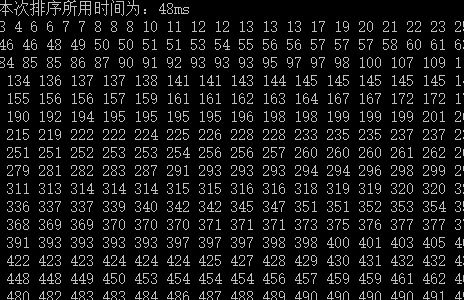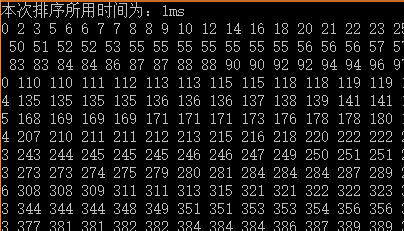# 认识堆排序的排序过程

## 1，实用的排序算法：选择排序

（1）选择排序的基本思想是：每一趟（例如第i趟，i=0，1，2，3，……n-2）在后面n-i个待排序元素中选择排序码最小的元素，作为有序元素序列的第i个元素。待到第n-2趟做完，待排序元素只剩下一个，就不用再选了。
（2）三种常用的选择排序方法
1>直接选择排序
2>锦标赛排序
3>堆排序

（3）直接选择排序简介
1>直接选择排序（select sort）是一种简单的排序方法，它的基本步骤是：
1）在一组元素V[i]~V[n-1]中选择具有最小排序码的元素；
2）若它不是这组元素中的第一个元素，则将它与这组元素中的第一个元素对调；
3）在这组元素中剔除这个具有最小排序码的元素，在剩下的元素V[i+1]~V[n-1]中重复执行1、2步骤，直到剩余元素只有一个为止。
2>直接选择排序使用注意

3>直接选择排序C++函数代码

//函数功能，直接选择排序算法对数列排序
//函数参数，数列起点，数列终点
void dselect_sort(const int start, const int end) {
for (int i = start; i < end; ++i) {
int min_position = i;
for (int j = i + 1; j <= end; ++j) {    //此循环用来寻找最小关键码
if (numbers[j] < numbers[min_position]) {
min_position = j;
}
}
if (min_position != i) {    //避免自己与自己交换
swap(numbers[min_position], numbers[i]);
}
}
}

（4）关于锦标赛排序

## 2，堆排序的排序过程

（1）个人理解：堆排序是选择排序的一种，所以它也符合选择排序的整体思想。直接选择排序是在还未成序的元素中逐个比较选择，而堆排序是首先建立一个堆（最大堆或最小堆），这使得数列已经“大致”成序，之后只需要局部调整来重建堆即可。建立堆及重建堆这一过程映射到数组中，其实就是一个选择的过程，只不过不是逐个比较选择，而是借助完全二叉树来做到有目的的比较选择。这也是堆排序性能优于直接选择排序的一个体现。
（2）堆排序分为两个步骤
1>根据初始输入数据，利用堆的调整算法形成初始堆；
2>通过一系列的元素交换和重新调整堆进行排序。
（3）堆排序的排序思路
1>前提，我们是要对n个数据进行递增排序，也就是说拥有最大排序码的元素应该在数组的末端。
2>首先建立一个最大堆，则堆的第一个元素heap具有最大的排序码，将heap与heap[n-1]对调，把具有最大排序码的元素交换到最后，再对前面n-1个元素，使用堆的调整算法siftDown(0,n-2)，重新建立最大堆。结果具有次最大排序码的元素又浮到堆顶，即heap的位置，再对调heap与heap[n-2]，并调用siftDown(0，n-3)，对前n-2个元素重新调整，……如此反复，最后得到一个数列的排序码递增序列。
（4）堆排序的排序过程

//函数功能，自上向下比较将一个集合局部调整为最大堆
//函数参数，调整起始节点，调整最终节点
void max_heap::siftDown(const int start,const int end) {
int i = start;
int j = 2 * i + 1;  //起始节点的左子树
int temp = heap[i];
while (j <= end) {  //确定temp最终的位置
if (j < end && heap[j] < heap[j + 1]) { //定位到左右子树的较大者
++j;
}
if (heap[j] <= temp) {  //与当前根值比较
break;
}
else {
heap[i] = heap[j];
i = j;
j = j * 2 + 1;
}
}
heap[i] = temp;
}

//函数功能，堆排序算法对数列递增排序
//函数参数，NULL
void max_heap::heap_sort() {
for (int cnt = currentSize - 1; cnt > 0; --cnt) {
swap(heap, heap[cnt]);
siftDown(0, cnt - 1);   //重新调整为最大堆
}
}

（5）构建堆与堆排序的关系

## 3，堆排序算法的性能

（1）通过数学分析可以得出堆排序的时间复杂度是O(nlog2(n))，并且在调整堆的过程中只借助了一个临时存储变量temp，所以其空间复杂度是O(1)。
（2）堆排序借助“堆”这个抽象数据类型实现元素的选择，这使得它比直接选择排序的效率更高。下面我们通过1000个随机数据（范围<1000）的排序，从两种排序算法的执行时间上认识它们的性能。

int main()
{
////直接选择排序测试
//time_t c_start, c_end;
//produceRandomNumbers(1, 1000, 1000);

//c_start = clock();
//dselect_sort(0, 999);
//c_end = clock();

//cout << "本次排序所用时间为：" << difftime(c_end, c_start) << "ms" << endl;
//for (auto value : numbers) {
//  cout << value << " ";
//}

//堆排序测试
time_t c_start, c_end;
randomNumbersToArray(1, 1000, 999);

c_start = clock();
max_heap heap(data3, 1000);
heap.heap_sort();
c_end = clock();

cout << "本次排序所用时间为：" << difftime(c_end, c_start) << "ms" << endl;
heap.display();

system("pause");
return 0;
}

（3）打印测试结果：（注：两次的测试数据并不同，但是通过多次随机数测试，其值几乎在本次测试附近）

/*
* 抽象数据类型——最大堆
*/
#include <iostream>
using namespace std;

const int DefaultSize = 10;

class max_heap {
int* heap;
int currentSize;
int maxSize;
void siftDown(const int start,const int end);
public:
max_heap(int sz = DefaultSize);
max_heap(int* h, int sz);
~max_heap();
void heap_sort();
void display() const;
};

//成员函数定义
max_heap::max_heap(int sz) {
heap = new int[sz];
if (!heap) {
cerr << "内存分配失败" << endl;
exit(EXIT_FAILURE);
}
currentSize = 0;
maxSize = (sz <= DefaultSize) ? DefaultSize : sz;
}

max_heap::max_heap(int* h, int sz) {
heap = new int[sz];
if (!heap) {
cerr << "内存分配失败" << endl;
exit(EXIT_FAILURE);
}
//初始化成员变量
currentSize = sz;
maxSize = (sz <= DefaultSize) ? DefaultSize : sz;
for (int cnt = 0; cnt < sz; ++cnt) {
heap[cnt] = h[cnt];
}
//调整为最大堆
int currentPosition = (currentSize - 2) / 2;    //最后一个分支节点——调整起点
while (currentPosition >= 0) {
siftDown(currentPosition--, currentSize - 1);
}
}

max_heap::~max_heap() {
delete[] heap;
}

//函数功能，自上向下比较将一个集合局部调整为最大堆
//函数参数，调整起始节点，调整最终节点
void max_heap::siftDown(const int start,const int end) {
int i = start;
int j = 2 * i + 1;  //起始节点的左子树
int temp = heap[i];
while (j <= end) {  //确定temp最终的位置
if (j < end && heap[j] < heap[j + 1]) { //定位到左右子树的较大者
++j;
}
if (heap[j] <= temp) {  //与当前根值比较
break;
}
else {
heap[i] = heap[j];
i = j;
j = j * 2 + 1;
}
}
heap[i] = temp;
}

//函数功能，堆排序算法对数列递增排序
//函数参数，NULL
void max_heap::heap_sort() {
for (int cnt = currentSize - 1; cnt > 0; --cnt) {
swap(heap, heap[cnt]);
siftDown(0, cnt - 1);   //重新调整为最大堆
}
}

void max_heap::display() const{
for (int cnt = 0; cnt < currentSize; ++cnt) {
cout << heap[cnt] << " ";
}
}

#endif

（注：以上代码可以放在自己命名（如：max_heap.h）的头文件中）

## 5，参考资料及引用

http://blog.csdn.net/weixin_37818081/article/details/78685916

08-046万+
06-204万+12-14635
08-2243万+
08-252690
05-241万+
04-201万+
11-083694
04-123022
09-022283